# Discount And Commission

##### Class 8th Mathematics (new) MHB Solution

###### Practice Set 9.1
Question 1.

If marked price = Rs 1700, selling price = Rs 1540 then find the discount.

Given:

Marked Price = Rs 1700

Selling Price = Rs 1540

Now, we know Discount = Marked Price – Selling Price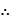Discount = 1700 – 1540 = Rs 160

Hence, the discount is Rs 160.

Question 2.

If marked price = Rs 990 and percentage of discount is 10, then find the selling price.

Given:

Marked Price = Rs 990

Discount = 10%

Now, let us find the ratio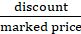Let us assume we obtain discount Rs x on the marked price.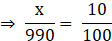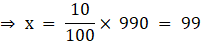So, Discount = Rs 99.

We know Selling Price = Marked Price – DiscountSelling Price = 990 – 99 = Rs 891

Hence, the selling price is Rs 891.

Question 3.

If selling price = Rs 900. Discount is 20 %, then find the marked price.

Given:

Selling Price = Rs 900

Discount = 20%

Now, let us suppose the marked price is Rs x.

Given discount is 20% on the marked price.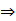Discount = 0.2x

We know Selling Price = Marked Price – Discount900 = x – 0.2x900 = 0.8x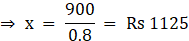Hence, the marked price is Rs 1125.

Question 4.

The marked price of the fan is 3000 rupees. Shopkeeper gave 12% discount on it. Find the total discount and selling price of the fan.

Given:

Marked Price = Rs 3000

Discount = 12%

Given discount is 12% on the marked price.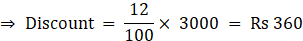Hence, the discount is Rs 360.

We know Selling Price = Marked Price – DiscountSelling Price = 3000 – 360 = Rs 2640

Hence, the selling price is Rs 2640.

Question 5.

The marked price of a mixer is 2300 rupees. A customer purchased it for Rs.1955. Find percentage of discount offered to the customer.

Given:

Marked Price = Rs 2300

Selling Price = Rs 1955

We know Discount = Marked Price – Selling PriceDiscount = 2300 – 1955 = Rs 345

Now, let us suppose the percentage of discount is x.

Then, we have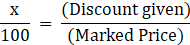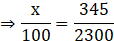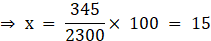Hence, the percentage of discount offered is 15 (that is 15%).

Question 6.

A shopkeeper gives 11% discount on a television set, hence the cost price of it is Rs. 22,250. Then find the marked price of the television set.

Given:

Discount = 11%

Cost Price = Rs 22250

Now, let us suppose the marked price is Rs x.

Given discount is 11% on the marked price.Discount = 0.11x

We also know the cost price is Rs 22250 after a discount of 11% on the marked price.x – 0.11x = 222500.89x = 22250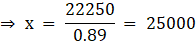Hence, the marked price is Rs 25000.

Question 7.

After offering discount of 10% on marked price, a customer gets total discount of 17 rupees. To find the cost price for the customer, fill in the following boxes with appropriate numbers and complete the activity.

Suppose, marked price of the item = 100 rupees

Therefore, for customer that item costs
[ ] - [ ] = 90 rupees

Hence, when the discount is [ ] then the selling price is [ ] rupees.

Suppose when the discount is [ ] rupees, the selling price is x rupees.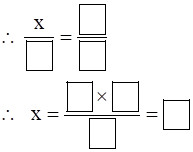∴ the customer will get the item for 153 rupees.

Suppose, marked price of the item = 100 rupees

Therefore, for customer that item costs
 -  = 90 rupees

(Given the discount is 10% on the marked price.Discount = 0.10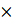100 = 10 rupees

Also, we have Cost Price = Marked Price - DiscountCost Price = 100 – 10 = 90 rupees)

Hence, when the discount is  rupees then the selling price is  rupees.

Suppose when the discount is  rupees, the selling price is x rupees (as it is given that customer gets a 17 Rupees discount).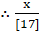=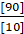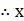=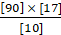= 

∴ the customer will get the item for 153 rupees.

Question 8.

A shopkeeper decides to sell a certain item at a certain price. He tags the price on the item by increasing the decided price by 25%. While selling the item, he offers 20% discount. Find how many more or less percent he gets on the decided price.

Let us assume the decided price is Rs 100.

Given the marked price is 25% more than decided price.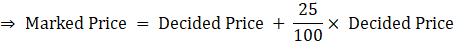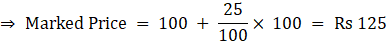Also given that discount is 20% on the marked price.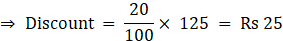We know Selling Price = Marked Price – DiscountSelling Price = 125 – 25 = Rs 100

So, when the decided price is Rs 100, the selling price is Rs 100.

Therefore, the shopkeeper sold the item for 0% more than the decided price (neither any profit nor any loss).

###### Practice Set 9.2
Question 1.

John sold books worth rupees 4500 for a publisher. For this he received 15% commission. Complete the following activity to find the total commission John obtained.

Selling price of books = [ ]

Rate of commission = [ ]

Commission obtained = [ ]/[ ] × [ ]

∴ Commission = [ ] rupees

Selling price of books = [Rs 4500]

Rate of commission = [15%]

(We have Commission = Commission RateSelling Price)

Commission obtained =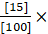∴ Commission =  rupees

Question 2.

Rafique sold flowers worth Rs 15,000 by giving 4% commission to the agent. Find the commission he paid. Find the amount received by Rafique.

Given:

Selling Price = Rs 15000

Commission rate = 4%

We have Commission = Commission RateSelling Price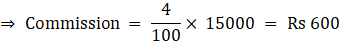Hence, Rafique paid a commission of Rs 600.

Amount received by Rafique = Selling Price – Commission

∴ Amount received = 15000 – 600 = Rs 14400

Hence, the amount received by Rafique is Rs 14400.

Question 3.

A farmer sold food grains for 9200 rupees through an agent. The rate of commission was 2%. How much amount did the agent get?

Given:

Selling Price = Rs 9200

Commission rate = 2%

We have Commission = Commission RateSelling Price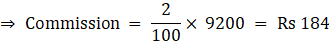Hence, agent got a commission of Rs 184.

Question 4.

Umatai purchased following items from a Khadi - Bhandar.

(i) 3 sarees for 560 rupees each.

(ii) 6 bottles of honey for 90 rupees each.

On the purchase, she received a rebate of 12%. How much total amount did Umatai pay?

Given:

Rebate = 15%

Cost of 3 sarees = 3560 = Rs 1680

Cost of 6 bottles = 690 = Rs 540

Hence, total cost of purchased items = 1680 + 540 = Rs 2220

Given rebate rate = 12%

We have Rebate = Rebate RateCost Price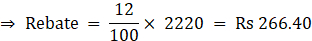Amount payed by Umatai = Total cost – Rebate

∴ Amount payed = 2220 – 266.40 = Rs 1953.60

Hence, the total amount payed by Umatai is Rs 1953.60.

Question 5.

Use the given information and fill in the boxes with suitable numbers.

Smt. Deepanjali purchased a house for Rs 7,50,000 from Smt. Leelaben through an agent. Agent has charged 2% brokerage from both of them.

(1) Smt. Deepanjali paid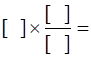Rs
[ ] brokerage for purchasing the house.

(2) Smt. Leelaben paid brokerage of Rs [ ].

(3) Total brokerage received by the agent is Rs [ ].

(4) The cost of house Smt. Deepanjali paid is Rs [ ].

(5) The selling price of house for Smt. Leelaben is Rs [ ].

Smt. Deepanjali purchased a house for Rs 7,50,000 from Smt. Leelaben through an agent. Agent has charged 2% brokerage from both of them.

(1)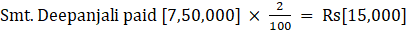brokerage for purchasing the house.

(We have Commission = Commission RateSelling Price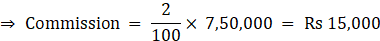(2) Smt. Leelaben paid brokerage of Rs [15,000]

(since the agent has charged equal brokerage from both of them).

(3) Total brokerage received by the agent is Rs [30,000].

(4) The cost of house Smt. Deepanjali paid is Rs [7,65,000].

(Amount Deepanjali paid = Selling Price + BrokerageAmount paid = 7,50,000 + 15,000 = Rs 7,65,000)

(5) The selling price of house for Smt. Leelaben is Rs [7,35,000].

(Effective Selling Price = Actual Selling Price – BrokerageSelling price = 7,50,000 - 15,000 = Rs 7,35,000)

## PDF FILE TO YOUR EMAIL IMMEDIATELY PURCHASE NOTES & PAPER SOLUTION. @ Rs. 50/- each (GST extra)

SUBJECTS

HINDI ENTIRE PAPER SOLUTION

MARATHI PAPER SOLUTION
SSC MATHS I PAPER SOLUTION
SSC MATHS II PAPER SOLUTION
SSC SCIENCE I PAPER SOLUTION
SSC SCIENCE II PAPER SOLUTION
SSC ENGLISH PAPER SOLUTION
SSC & HSC ENGLISH WRITING SKILL
HSC ACCOUNTS NOTES
HSC OCM NOTES
HSC ECONOMICS NOTES
HSC SECRETARIAL PRACTICE NOTES

2019 Board Paper Solution

HSC ENGLISH SET A 2019 21st February, 2019

HSC ENGLISH SET B 2019 21st February, 2019

HSC ENGLISH SET C 2019 21st February, 2019

HSC ENGLISH SET D 2019 21st February, 2019

SECRETARIAL PRACTICE (S.P) 2019 25th February, 2019

HSC XII PHYSICS 2019 25th February, 2019

CHEMISTRY XII HSC SOLUTION 27th, February, 2019

OCM PAPER SOLUTION 2019 27th, February, 2019

HSC MATHS PAPER SOLUTION COMMERCE, 2nd March, 2019

HSC MATHS PAPER SOLUTION SCIENCE 2nd, March, 2019

SSC ENGLISH STD 10 5TH MARCH, 2019.

HSC XII ACCOUNTS 2019 6th March, 2019

HSC XII BIOLOGY 2019 6TH March, 2019

HSC XII ECONOMICS 9Th March 2019

SSC Maths I March 2019 Solution 10th Standard11th, March, 2019

SSC MATHS II MARCH 2019 SOLUTION 10TH STD.13th March, 2019

SSC SCIENCE I MARCH 2019 SOLUTION 10TH STD. 15th March, 2019.

SSC SCIENCE II MARCH 2019 SOLUTION 10TH STD. 18th March, 2019.

SSC SOCIAL SCIENCE I MARCH 2019 SOLUTION20th March, 2019

SSC SOCIAL SCIENCE II MARCH 2019 SOLUTION, 22nd March, 2019

XII CBSE - BOARD - MARCH - 2019 ENGLISH - QP + SOLUTIONS, 2nd March, 2019

HSC Maharashtra Board Papers 2020

(Std 12th English Medium)

HSC ECONOMICS MARCH 2020

HSC OCM MARCH 2020

HSC ACCOUNTS MARCH 2020

HSC S.P. MARCH 2020

HSC ENGLISH MARCH 2020

HSC HINDI MARCH 2020

HSC MARATHI MARCH 2020

HSC MATHS MARCH 2020

SSC Maharashtra Board Papers 2020

(Std 10th English Medium)

English MARCH 2020

HindI MARCH 2020

Hindi (Composite) MARCH 2020

Marathi MARCH 2020

Mathematics (Paper 1) MARCH 2020

Mathematics (Paper 2) MARCH 2020

Sanskrit MARCH 2020

Important-formula

THANKS